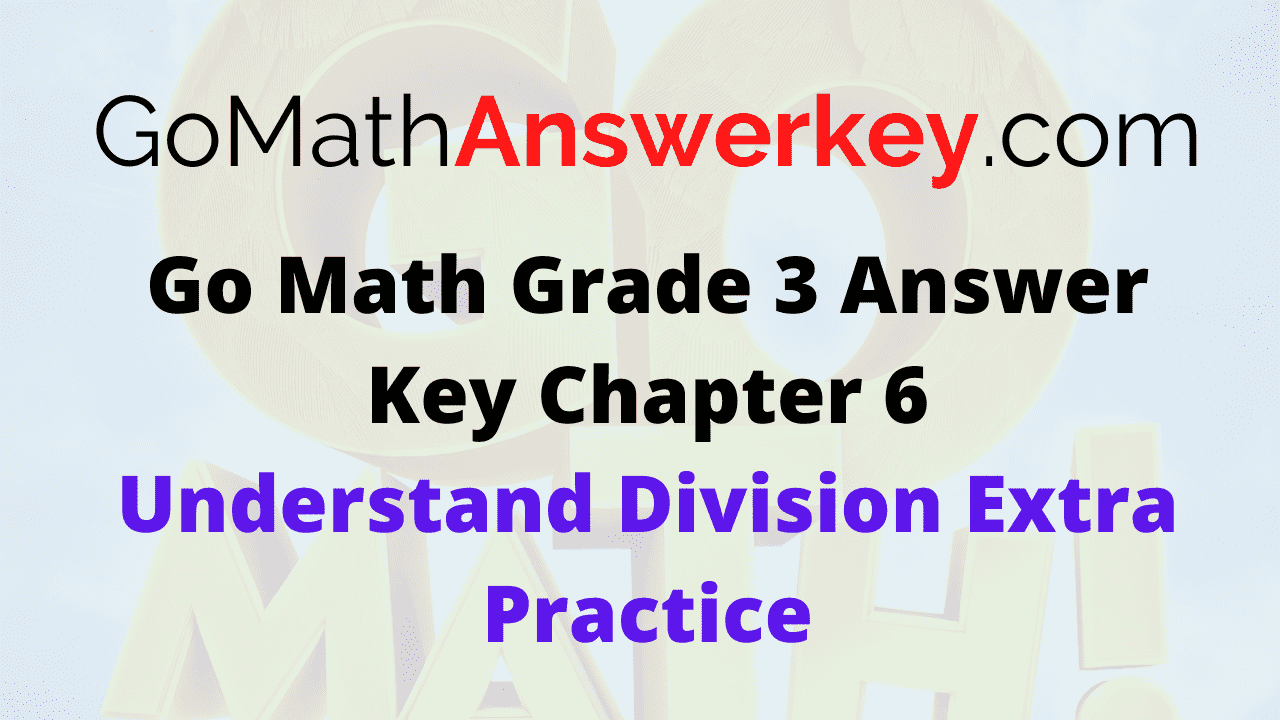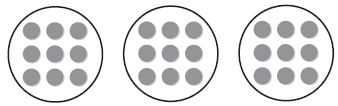# Go Math Grade 3 Answer Key Chapter 6 Understand Division Extra Practice

Students who are willing to practice more questions can Download Go Math Grade 3 Answer Key Chapter 6 Understand Division Extra Practice. It is very important to learn the basics or fundamentals to become master in maths. Students of Grade 3 can easily understand the basics of division provided in the Go Math Grade 3 Answer Key Chapter 6 Understand Division Extra Practice.

## Go Math Grade 3 Answer Key Chapter 6 Understand Division Extra Practice

The topics covered in this chapter are Repeated subtraction, Equal groups, Number line, related multiplication, and division facts. Check the topics and start your preparation for the exam. Get the step by step procedure to understand Division.

### Common Core – Page No. 123000

Lessons 6.1–6.3 Make equal groups.

Complete the table.

 Counters Number of Equal Groups Number in Each Group 1. 18 9 ________ 2. 24 ________ 8 3. 12 6 ________ 4. 35 7 ________ 5. 32 ________ 4 6. 25 ________ 5

 Counters Number of Equal Groups Number in Each Group 1. 18 9 2 2. 24 3 8 3. 12 6 2 4. 35 7 5 5. 32 8 4 6. 25 5 5

Explanation:

1. Number of counters = 18
Number of equal groups = 9
Number in each group = x
x × 9 = 18
x= 18/9 = 2
Therefore number in each group = 2

2. Number of counters = 24
Number in each group = 8
Number of equal groups = x
x × 8 = 24
x = 24/8 = 3
Thus the number of equal groups = 3

3. Number of counters = 12
Number of equal groups = 6
Number in each group = x
x × 6 = 12
x = 12/6 = 2
So, the number in each group = 2

4. Number of counters = 35
Number of equal groups = 7
Number in each group = x
x × 7 = 35
x = 35/7 = 5
x = 5
Therefore number in each group = 5

5. Number of counters = 32
Number of equal groups = x
Number in each group = 8
x × 8 = 32
x = 32/8 = 4
Thus the number of equal groups = 4

6. Number of counters = 25
Number of equal groups = x
Number in each group = 5
x × 5 = 25
x = 25/5 = 5
So, the number of equal groups = 5

Lesson 6.4

Write a division equation for the picture.

Question 1.Type below:
__________

Answer: 27 ÷ 3 = 9 or 27 ÷ 9 = 3

Explanation:

Total number of counters = 27
Number of equal groups = 3
Number in each group = 9
The division equation is
Number of counters by number of groups = 27 ÷ 3 = 9
or
Number of counters by number in each group = 27 ÷ 9 = 3

Question 2.Type below:
__________

Answer: 24 ÷ 4 = 6 or 24 ÷ 6 = 4

Explanation:

Total number of counters = 24
Number of equal groups = 4
Number in each group = 6
The division equation is
Number of counters by number of groups = 24 ÷ 4 = 6
or
Number of counters by number in each group = 24 ÷ 6 = 4

Lesson 6.5

Write a division equation.

Question 3.Type below:
__________

Answer: 3 groups, 15 ÷ 5 = 3

Explanation:

Step 1:

Starts at 15

Step 2:

Count back by 5s as many times as you can.

Step 3:

Count the number of times you jumped back 5.
You jumped back by 15 three times
There are 3 jumps of 5 in 15.

Question 4.Type below:
__________

Answer: 24 ÷ 6 = 4

Explanation:

Step 1:

Begins at 24

Step 2:

Subtract with 6 until you get 0.

Step 3:

Count the number of times you subtract with 6.

You subtract 4 times
There are 4 groups of 6 with 24
So, 24 ÷ 6 = 4

### Common Core – Page No. 124000

Lesson 6.6

Make an array. Then write a division equation.

Question 1.
12 tiles in 4 rows
______ ÷ ______ = ______

Answer: 12 ÷ 4 = 3

Explanation:

■ ■ ■
■ ■ ■
■ ■ ■
■ ■ ■
Total number of tiles = 12
Number of rows = 4
Number of tiles in each row = x
Divide the number of tiles by number of rows = 12 ÷ 4 = 3

Question 2.
18 tiles in 3 rows
______ ÷ ______ = ______

Answer: 18 ÷ 3 = 6

Explanation:

■ ■ ■ ■ ■ ■
■ ■ ■ ■ ■ ■
■ ■ ■ ■ ■ ■
Total number of tiles = 18
Number of rows = 3
Number of tiles in each row = y
Divide the number of tiles by no. of rows = 18 ÷ 3 = 6

Question 3.
35 tiles in 5 rows
______ ÷ ______ = ______

Answer: 35 ÷ 5 = 7

Explanation:

■ ■ ■ ■ ■ ■ ■
■ ■ ■ ■ ■ ■ ■
■ ■ ■ ■ ■ ■ ■
■ ■ ■ ■ ■ ■ ■
■ ■ ■ ■ ■ ■ ■

Total number of tiles = 35
Number of rows = 5
Number of tiles in each row = p
Divide the number of tiles by number of rows = 35 ÷ 5 = 7

Question 4.
28 tiles in 7 rows
______ ÷ ______ = ______

Answer: 28 ÷ 7 = 4

Explanation:

■ ■ ■ ■
■ ■ ■ ■
■ ■ ■ ■
■ ■ ■ ■
■ ■ ■ ■
■ ■ ■ ■
■ ■ ■ ■

Total number of tiles = 28
Number of rows = 7
Number of tiles in each row = x
Divide the number of tiles by number of rows = 28 ÷ 7 = 4

Lesson 6.7

Complete the equations.

Question 5.
8 × ______ = 40 40 ÷ 8 = ______

Explanation:

Let x be the unknown factor
8 × x = 40
x = 40/8
x = 5
Check whether the related multiplication and division facts are the same or not.
40 ÷ 8 = 5
The related facts of 40 and 8 are 5.

Question 6.
6 × ______ = 36 36 ÷ 6 = ______

Explanation:

Let y be the unknown factor
6 × y = 36
y = 36/6 = 6
Check if the related multiplication and division facts are the same or not.
36 ÷ 6 = 6
The related facts of 36 and 6 are 6.

Question 7.
3 × ______ = 21 21 ÷ 3 = ______

Explanation:

Let x be the unknown factor
3 × x = 21
x = 21
Check whether the related facts are the same or not.
21 ÷ 3 = 7
The quotient is 7.

Question 8.
2 × ______ = 18 18 ÷ 2 = ______

Explanation:

Let b be the unknown factor
2 × b = 18
b = 18/2 = 9
Check the related multiplication and division facts
18 ÷ 2 = 9
The related facts of 18 and 2 are 9.

Lesson 6.8 (pp. 239–243)

Write the related facts for the array.

Question 9.
■ ■ ■ ■ ■
■ ■ ■ ■ ■
■ ■ ■ ■ ■
______ × ______ = ______
______ × ______ = ______
______ ÷ ______ = ______
______ ÷ ______ = ______

3 × 5 = 15
5 × 3 = 15
15 ÷ 3 = 5
15 ÷ 5 = 3

Explanation:

Total number of tiles = 15
Number of equal rows = 3
Number of rows in each group = 5
So, the related 5, 3 and 15 is 5× 3 = 15, 3×5 = 15, 15 ÷ 3 = 5 and 15÷ 5 = 3

Question 10.
■ ■ ■ ■ ■ ■
■ ■ ■ ■ ■ ■
■ ■ ■ ■ ■ ■
______ × ______ = ______
______ × ______ = ______
______ ÷ ______ = ______
______ ÷ ______ = ______

3 × 6 = 18
6 × 3 = 18
18 ÷ 3 = 6
18 ÷ 6 = 3

Explanation:

Total number of tiles = 18
Number of equal rows = 3
Number of rows in each group = 6
So, the related 18, 3 and 6 is 3 × 6 = 18, 6 × 3 = 18, 18 ÷ 3 = 6 and 18 ÷ 6 = 3

Question 11.
■ ■ ■ ■ ■
■ ■ ■ ■ ■
______ × ______ = ______
______ × ______ = ______
______ ÷ ______ = ______
______ ÷ ______ = ______

2 × 5 = 10
5 × 2 = 10
10 ÷ 2 = 5
10 ÷ 5 = 2

Explanation:

Total number of tiles = 10
Number of equal rows = 2
Number of rows in each group = 5
So, the related 2, 5 and 10 is 2 × 5 = 10, 5 × 2 = 10, 10 ÷ 2 = 5 and 10 ÷ 5 = 2

Lesson 6.9

Find the quotient.

Question 12.
7 ÷ 1 = ______

Explanation:

Any number divided by 1 will be the same number. Thus the quotient is 7.

Question 13.
4 ÷ 4 = ______

Explanation:

The number divided by the same number will be always 1. Thus the quotient is 1.

Question 14.
9 ÷ 1 = ______

Explanation:

Any number divided by 1 will be always the same number. So, the quotient is 9.

Question 15.
0 ÷ 1 = ______

Explanation:

0 divided by any number is always 0. So, the quotient is 0.

Question 16.
Anton has 8 flower pots. He plants 1 seed in each pot. How many seeds does Anton use?
______ seeds

Explanation:

Anton has 8 flower pots.
He plants 1 seed in each pot.
Number of seeds Anton used = x
x × 1 = 8
x = 8/1
x = 8
Therefore there are 8 seeds in 8 flower pots.

The problems in the Go Math Grade 3 Answer Key Chapter 6 Understand Division Extra Practice helps the students to learn the concepts and for exam preparation. If you have any doubts in this chapter you can refer Go Math Grade 3 Answer Key Chapter 6 Understand Division. The students of Grade 3 can get all chapters solution in our Go Math Answer Key.

Scroll to Top